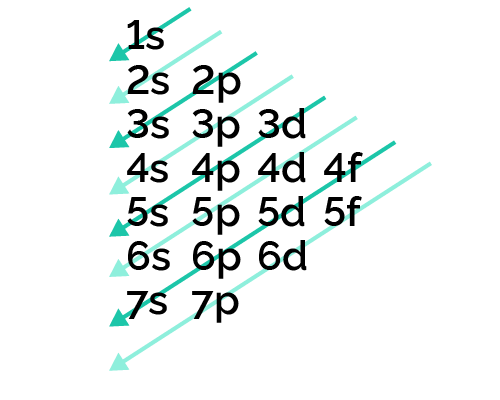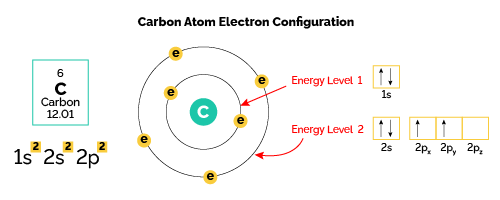# Electron Configuration Calculator

Created by Joanna Śmietańska, PhD candidate
Reviewed by Łucja Zaborowska, MD, PhD candidate and Rijk de Wet
Last updated: Jun 05, 2023

This electron configuration calculator will instantly show you the distribution of electrons in the orbitals of any periodic element you choose. Typically, you need at least 8 steps to determine the electron configuration, starting with finding the atomic number by looking at the list of orbitals and understanding the notation.

But wait — you can avoid all this if you use our tool! Save time and effort by simply selecting the name of the element, and you'll get the complete electron configuration, along with the atomic number and mass of all 118 known elements. What's more, this tool also works as a valence electron calculator and gives the valence electrons of all the elements of the periodic table.

Are you ready to excel in your science course? Read on to find out what an electron configuration is, what valence electrons are, and how to find valence electrons of any element.

## Ground state electron configuration

Electron configuration notation provides us with information about the basic energy levels and sublevels that electrons occupy. Ground state means that the atom has the lowest energy allowed. The electron configuration is responsible for many physical and chemical properties of an element. Let us look at the electron configuration for helium, which is $1{\rm s}^2$.

• The first integer, $1$, indicates the primary energy level. In general, its values can be between $1$ to $n$, where $n$ is the value of the outermost shell containing an electron.

• The letter $\rm s$ indicates the type of orbital (a.k.a. the subshell). Different subshells can host a different number of electrons: subshell $\rm s$ has a maximum of $2$ electrons, subshell $\rm p$ has up to $6$ electrons, subshell $\rm d$ up to $10$ electrons, and subshell $\rm f$ up to $14$ electrons.

• The superscript 2 ($^2$) tells you the electron occupancy on the corresponding subshell.

## Electron configuration chart and Hund's rule

To write down the electron configuration of a given atom, we use general rules:

• The aufbau principle (from German "aufbau" meaning "building up") states that electrons always occupy orbitals of increasing energy, so the order in which the orbitals are occupied follows the electron configuration chart below:The order is:

1s ← 2s ← 2p ← 3s ← 3p ← 4s ← 3d ← 4p ← 5s ← 4d ← 5p ← 6s ← 4f ← 5d ← 6p ← 7s ← 5f ← 6d ← 7p

• Hund's rule states that an atom in the ground state always has as many unpaired electrons as possible. This means to say that electron pairs only form after all orbital levels have been filled by unpaired electrons. For example, the ground state electron configuration of nitrogen ($\rm 1s^22s^22p^3$) indicates that it has $3$ electrons occupying the $2 \rm p$ orbital. Based on Hund's rule, one electron fills each $\rm p$ orbital, and each electron has the same spin. Briefly, the electron spin determines whether the atom will generate a magnetic field. Its values are limited to $+1/2$ (upward arrow) or $-1/2$ (downward arrow). If the spins are paired, no magnetic field will appear, but one unpaired electron will determine the spin.

• Pauli exclusion principle means that if two electrons occupy the same orbitals their spins must be paired, as indicated by the direction of the arrows (↑) and (↓).

Let's combine these rules to write down the electron configuration of carbon with an atomic number of 6 (meaning it contains 6 electrons in its ground state).According to the electron configuration chart, we will put 6 electrons in the following orbitals:

$\mathrm{C} = \rm 1s^22s^22p^2$

The $\rm 1s$ and $\rm 2s$ orbitals are successively fully filled with two electrons. Now the next two electrons will enter the $2p$ orbital in an anti-clockwise direction, as can be seen in the diagram of the carbon orbitals.

## How to write electron configuration using shorthand method?

Chemists have found a method of writing the electron configuration more simply using the shorthand method. Let us return to the electron configuration of nitrogen and write it again:

1. Find the atomic number of nitrogen (7) and use this electron configuration calculator to get a complete electron configuration. Otherwise, write the order of the energy levels with electron configuration chart: $\rm 1s^22s^22p^3$.

2. Put the brackets around the electron configuration of the last noble gas, group 18 element, before nitrogen. Note that helium (He) is the noble gas preceding nitrogen.

3. Continue with the electron configuration after helium. So for nitrogen, it would look like this: $[{\rm He}]\rm 2s^22p^3$

Similarly, we can use the shorthand method to write the electron configuration of carbon, keeping in mind that it will have 1 less electron on the $2p$ shell than helium, hence $[{\rm He}]\rm 2s^22p^2$. This method works well for writing the electron configuration of any element.

## What are valence electrons?

The valence electrons (i.e., the electrons in the outermost subshell) have the highest energy. They are particularly important because they are involved in bond formation and determine the chemical properties of an element. Find out more about how the attraction of an electron to a nucleus will change with our effective nuclear charge calculator.

How to find the valence electrons of a chosen element? You can check it immediately in our valence electron calculator on the left or look at the position of a particular atom on the periodic table, which will help you to determine how many valence electrons a neutral atom of that element has. The group number that an element is in determines the number of its valence electrons.

For example, chlorine is in group 7, so it has seven valence electrons. Indeed, we can write its electron configuration as $\rm 1s^22s^22p^63s^23p^5$. As we can see, chlorine has seven electrons in the $\rm 3s$ and $\rm 3p$ orbitals, which are the valence electrons.

For groups 13 to 18, subtract 10 from the group number to get the number of valence electrons, e.g., elements from group 13 will have three valence electrons, from group 14 four valence electrons, and so on.

A notable case is group 18, in which the elements (known as noble gases) have 8 valence electrons. This is such a stable electron shell arrangement that they essentially have no valence electrons available for bonding or reacting.

## Examples of to use our electron configuration calculator

Let us use this smart electron configuration calculator to determine the electron configuration of copper:

1. Choose copper (Cu) from the drop-down list of elements.

2. Check the atomic number (29) and atomic mass (63.546) of copper. Read here how to calculate atomic mass.

3. Read the full electron configuration: $\rm 1s^2 2s^2 2p^6 3s^2 3p^6 3d^{10} 4s^1$.

Now you can say: "Wait a minute! According to the electron configuration chart and aufbau principle, should the electron configuration calculator not report it as $\rm 1s^2 2s^2 2p^6 3s^2 3p^6 3d^9 4s^2$? After all, we fill the $\rm 4s$ shell first and then the $\rm 3d$."

Well, in the copper atom, there is a disruption of the order of filling up the electron sub-shells, i.e., jumping one of the $\rm 4s^2$ electrons to the $\rm 3d^9$ is energetically more favorable. A similar phenomenon occurs in chromium and gold atoms.

1. Now use our tool as a valence electrons calculator and check the valence electrons of copper: $\rm 3d^{10} 4s^1$.

We know from the periodic table that copper is in group 11, so this agrees with the result of this electron configuration calculator.

## FAQ

### What is electron configuration?

An atom's ground state electron configuration describes how the electrons have distributed among the orbital shells and subshells. According to the electron configuration chart, electrons in an atom occupy orbitals according to their increasing energy, with each orbital having a maximum of two paired electrons with opposite spins.

### How can I write electron configuration of oxygen?

To find the electron configuration of oxygen:

1. Look at the periodic table and find an atomic number of oxygen, which is 8.
2. Fill these 8 electrons in the following order: 1s, 2s, and then 2p.
3. Write the complete electron configuration of oxygen: 1s²2s²2p⁴.
4. Identify the noble gas before oxygen, helium, and write using shorthand notation: [He]2s²2p⁴.

### How can I find electronic configuration for ions?

If an atom has lost or gained electrons by changing to a cation or anion, follow these steps:

1. Add an electron to the anion electron configuration. For example, the ground state electronic configuration of chlorine is 1s²2s²2p⁶3s²3p⁵. For Cl, it will be 1s²2s²2p⁶3s²3p⁶.

2. Remove the outermost electrons in the cation, e.g. electron configuration for Mg2+ will be 1s²2s²2p⁶.

### What element has the electron configuration of 1s2 2s2 2p6 3s2 3p4?

Sulfur (S). You can sum the number of electrons on all the subshells, and you will get the number 16. In its ground state, it corresponds to an atomic number that is unique to sulfur in the periodic table.

### How many valence electrons does carbon have?

4. The atomic number of carbon is 6, and the complete electronic configuration for this atom is 1s²2s²2p². You can see that in the periodic table, carbon is in group 4, so it has 4 valence electrons.

### How many valence electrons does oxygen have?

6. Oxygen is in group 16 of the periodic table, so as with the other elements in groups 13-18, you can subtract 10 from the group number to find out the number of valence electrons. Therefore, oxygen has 6 of them, two in the 2s subshell and four in the 2p subshell.

Joanna Śmietańska, PhD candidate
Element
Actinium (Ac)
Atomic number: 89
Atomic mass: 227
The electron configuration of element is:
1s2, 2s2, 2p6, 3s2, 3p6, 3d10, 4s2, 4p6, 4d10, 5s2, 5p6, 6s2, 4f14, 5d10, 6p6, 7s2, 6d1
The valence electrons are:
7s2, 6d1
People also viewed…

### Alien civilization

The alien civilization calculator explores the existence of extraterrestrial civilizations by comparing two models: the Drake equation and the Astrobiological Copernican Limits👽

### Grams to moles

Use this grams to moles calculator whenever you want to know the number of moles of a substance with a specific mass.

### Podcasts

Do you feel like you could be doing something more productive or educational while on a bus? Or while cleaning the house? Well, why don't you dive into the rich world of podcasts! With this podcast calculator, we'll work out just how many great interviews or fascinating stories you can go through by reclaiming your 'dead time'!

### Water hardness

Estimate the water hardness based on the calcium and magnesium concentration using the water hardness calculator.